# Online Civil Engineering Test - Structural Design Specifications TestLoading Test...

Instruction:

• This is a FREE online test. DO NOT pay money to anyone to attend this test.
• Total number of questions : 20.
• Time alloted : 30 minutes.
• Each question carry 1 mark, no negative marks.
• DO NOT refresh the page.
• All the best :-).

1.

For battened struts, the effective length is increased by

A.
 5%B.
 7.5%C.
 10%D.
 12.5%E.
 15%2.

The distance between, rivet line and the nearest edge of a joint not exposed to weather, is taken (where t is thickness in mm of the thinner outside plate).

A.
 6 tB.
 8 tC.
 10 tD.
 12 t3.

The effective and actual lengths of a cantilever are same if continuous at the support,

A.
 unstrained against torsion at the support and free at the endB.
 with partial restraint against torsion of the support and free at the endC.
 restrained against torsion at the support and free at the endD.
 none of the these.4.

In adverse circumtances, the reinforced concrete member immersed in sea water or subjected to sea spray, the maximum permissible cover for the reinforcing bars, should not exceed

A.
 40 mmB.
 50 mmC.
 60 mmD.
 70 mmE.
 75 mm5.

The minimum horizontal distance between two parallel main reinforcing bars, is

A.
 the diameter of the bar if their diameters are sameB.
 the diameter of the longer bar if their diameters are unequalC.
 5 mm more than nominal maximum size of the coarse aggragateD.
 greatest value of the above.6.

For concreting of heavily reinforced sections without vibration, the workability of concrete should be

A.
 very lowB.
 lowC.
 mediumD.
 high.7.

If b is actual width of a flange, Dt is the thickness of the flange, bω is the breath of the web, lo is the distance between the points of zero moments in a beam, the effective width of the flange, is

A.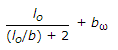B.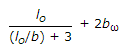C.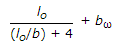D.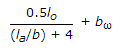8.

For calculating critical compression stress in bending Cs for sections symmetrical about the x-x axis, from the formula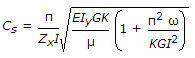, the value of G is taken

A.
 0.2 EB.
 0.3 EC.
 0.4 ED.
 0.5 E9.

For the proportions

A.
 1 :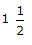the maximum size of aggregates, is 10 mmB.
 1 : 2 the maximum size of aggregates, is 20 mmC.
 1 :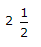the maximum size of aggregates, is 40 mmD.
 All the above.10.

Columns are designed for a minimum eccentricity equal to 1/30 (unsupported length ofthe column), subject to a minimum of

A.
 5 mmB.
 10 mmC.
 15 mmD.
 20 mm11.

Generally acceptable nominal size of aggregates for reinforced concrete, is

A.
 10 mmB.
 15 mmC.
 20 mmD.
 25 mmE.
 40 mm12.

As per IS : 800-1982, the coefficient of expansion for steel per degree centigrade per unit length, is

A.
 1.1e-05B.
 1.2e-05C.
 1.3e-05D.
 1.4e-0513.

The flat slab is a reinforced concrete slab with or without drops and is supported

A.
 on columns without column headB.
 on columns with column headC.
 on beamsD.
 None of these.14.

The individual part of the structures is subjected to a load test for 24 hours by applying a load equal to full dead load of the structure plus

A.
 imposed loadB.
 1.15 times imposed loadC.
 1.25 times imposed loadD.
 1.3 times imposed load.15.

The thickness of the rectangular plate for the cap or base of a solid round steel columns, is calculated from the formula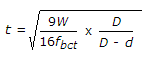where d is

A.
 the reduced diameter of the columnB.
 the dimension of the baseC.
 the diameter of the baseD.
 the diameter of the top.16.

The characteristic strength of concrete is the strength of material if its test result is not less than

A.
 2%B.
 3%C.
 4%D.
 5%E.
 10%17.

For nominal mix concrete M 20, the required quantity of water per 50 kg. of cement, is

A.
 60 litresB.
 45 litresC.
 34 litresD.
 30 litres18.

The development length of each bar of three bars bundled together is increased by

A.
 10%B.
 20%C.
 33%D.
 50%19.

As per IS : 1982, the permissible axial tension in rolled I-beams and channels, is

A.
 1500 kg/cm2B.
 1800 kg/cm2C.
 1400 kg/cm2D.
 1420 kg/cm220.

Minimum diameter of the lateral ties should not be less than

A.
 4 mmB.
 5 mmC.
 6 mmD.
 10 mm